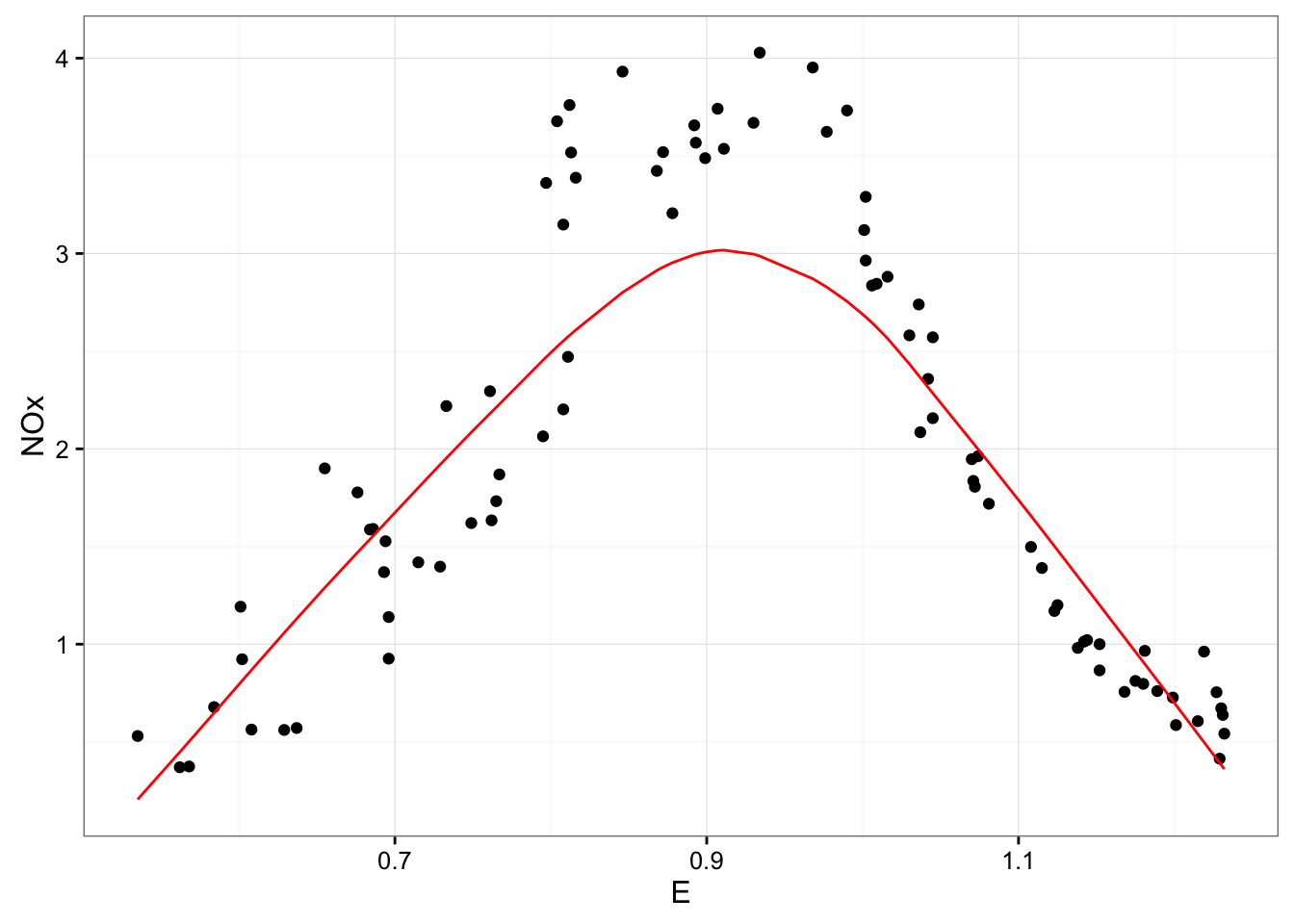Animations are excellent tools for teaching and building intuition. Here we show how a LOESS fit (with a 1-degree polynomial) is constructed, by performing a weighted regression in many overlapping windows.

First, we see how the broom package’s `augment` function is useful for plotting a LOESS fit.

``````library(lattice)
library(ggplot2)
library(broom)
theme_set(theme_bw())

mod <- loess(NOx ~ E, ethanol, degree = 1, span = .75)
fit <- augment(mod)

ggplot(fit, aes(E, NOx)) +
geom_point() +
geom_line(aes(y = .fitted), color = "red")``````We now need to compute a separate regression centered around each point on the graph. broom’s `inflate` function is useful for this, followed by some dplyr manipulation to filter the distant ones (using a span of .75). Notice that we filter out all but the 75% closest points, and then calculate tricube weights, just like the loess function does, on those points.

``````library(dplyr)

dat <- ethanol %>%
inflate(center = unique(ethanol\$E)) %>%
mutate(dist = abs(E - center)) %>%
filter(rank(dist) / n() <= .75) %>%
mutate(weight = (1 - (dist / max(dist)) ^ 3) ^ 3)

dat``````
``````## Source: local data frame [5,478 x 6]
## Groups: center 
##
##    center   NOx     C     E  dist     weight
##     (dbl) (dbl) (dbl) (dbl) (dbl)      (dbl)
## 1   0.535 3.741  12.0 0.907 0.372 0.38324129
## 2   0.535 2.295  12.0 0.761 0.226 0.82699305
## 3   0.535 1.498  12.0 1.108 0.573 0.00000000
## 4   0.535 2.881  12.0 1.016 0.481 0.06815599
## 5   0.535 3.120   9.0 1.001 0.466 0.09868125
## 6   0.535 2.358  12.0 1.042 0.507 0.02901265
## 7   0.535 3.669  12.0 0.930 0.395 0.30402352
## 8   0.535 1.192  18.0 0.601 0.066 0.99542253
## 9   0.535 0.926   7.5 0.696 0.161 0.93491730
## 10  0.535 1.590  12.0 0.686 0.151 0.94609661
## ..    ...   ...   ...   ...   ...        ...``````

We are now ready to create an animation, where each frame uses a different center. The most important layer here is the `geom_smooth` step with the `weight = weight` aesthetic. This does all the work of adding a best-fit line from the weighted regression. We also add a `geom_vline` to show the current center.

``````p <- ggplot(dat, aes(E, NOx)) +
geom_point(aes(alpha = weight, frame = center)) +
geom_smooth(aes(group = center, frame = center, weight = weight), method = "lm", se = FALSE) +
geom_vline(aes(xintercept = center, frame = center), lty = 2) +
geom_line(aes(y = .fitted), data = fit, color = "red")

library(gganimate)
gg_animate(p)``````